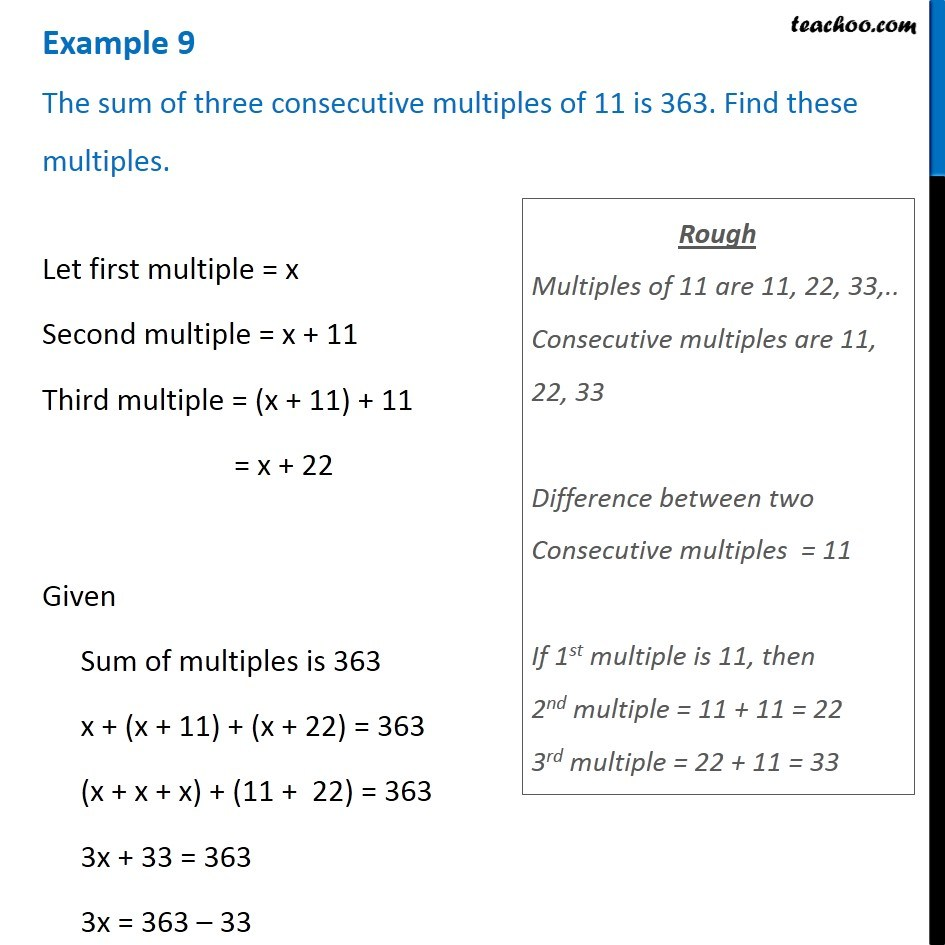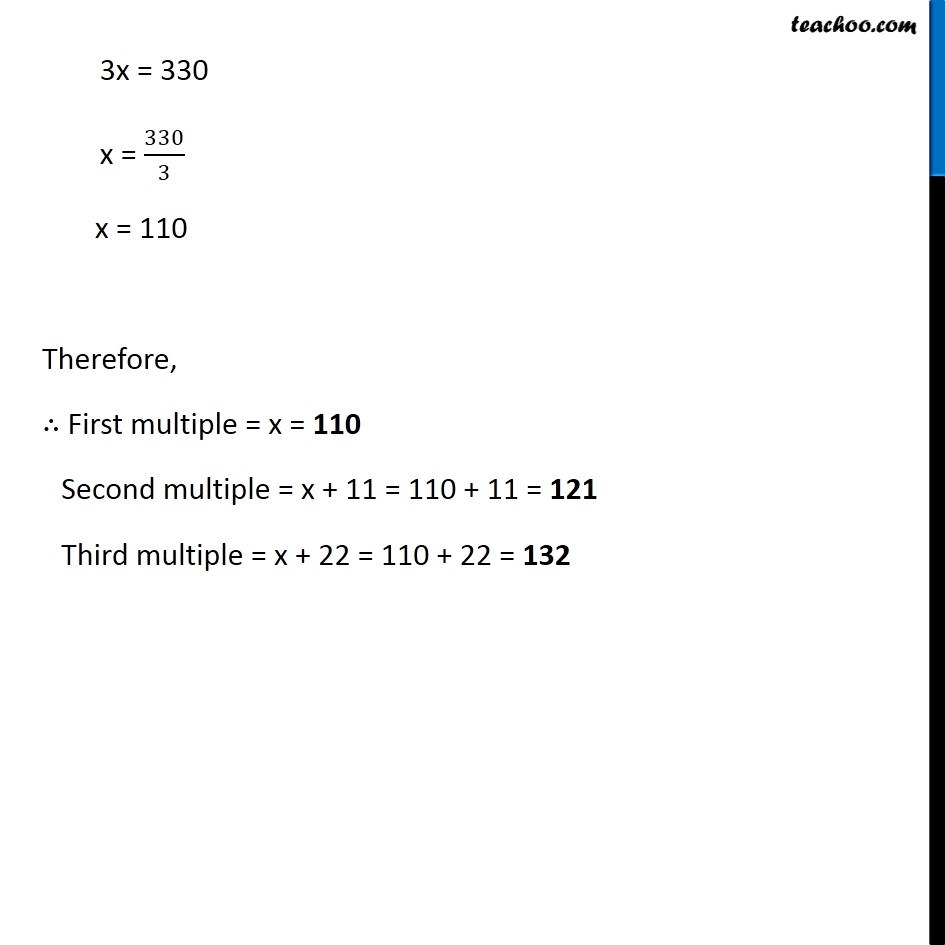Examples

Chapter 2 Class 8 Linear Equations in One Variable
Serial order wiseLearn in your speed, with individual attention - Teachoo Maths 1-on-1 Class

### Transcript

Question 9 The sum of three consecutive multiples of 11 is 363. Find these multiples.Let first multiple = x Second multiple = x + 11 Third multiple = (x + 11) + 11 = x + 22 Given Sum of multiples is 363 x + (x + 11) + (x + 22) = 363 (x + x + x) + (11 + 22) = 363 3x + 33 = 363 3x = 363 – 33 Rough Multiples of 11 are 11, 22, 33,.. Consecutive multiples are 11, 22, 33 Difference between two Consecutive multiples = 11 If 1st multiple is 11, then 2nd multiple = 11 + 11 = 22 3rd multiple = 22 + 11 = 33 3x = 330 x = 330/3 x = 110 Therefore, ∴ First multiple = x = 110 Second multiple = x + 11 = 110 + 11 = 121 Third multiple = x + 22 = 110 + 22 = 132